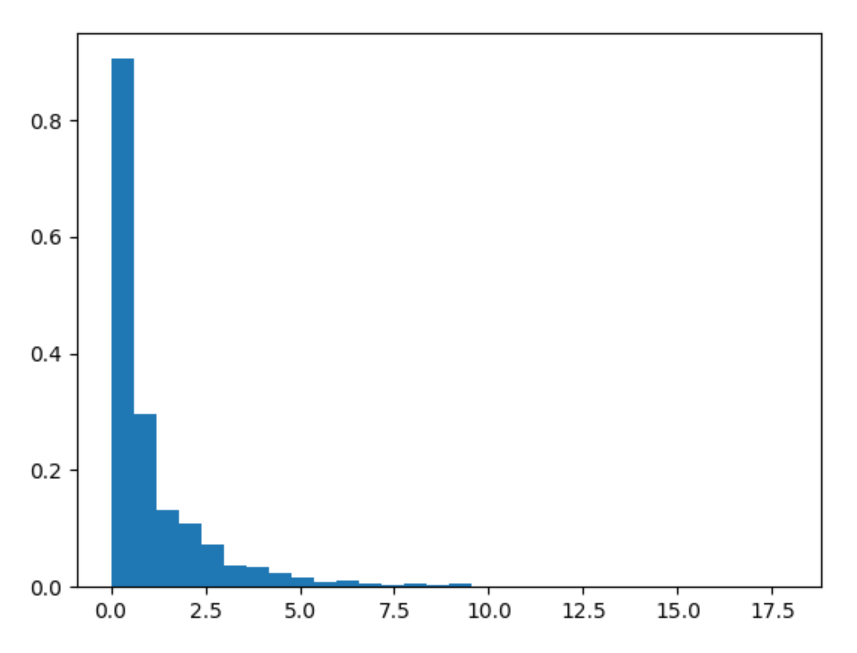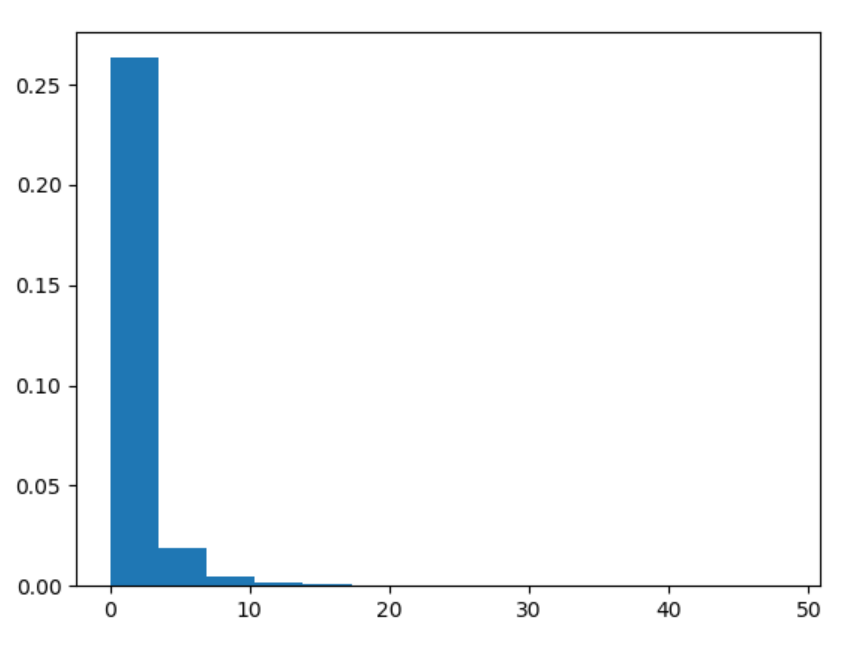# numpy.random.f() in Python

• Last Updated : 15 Jul, 2020

With the help of numpy.random.f() method, we can get the random samples of F distribution and return the random samples of numpy array by using this method.

Syntax : numpy.random.f(dfnum, dfden, size=None)

Return : Return the random samples as numpy array.

Example #1 :

In this example we can see that by using numpy.random.f() method, we are able to get the random samples of F distribution and return the random samples by using this method.

## Python3

 `# import numpy and f``import` `numpy as np``import` `matplotlib.pyplot as plt`` ` `# Using f() method``gfg ``=` `np.random.f(``0.98``, ``15.43``, ``1000``)`` ` `count, bins, ignored ``=` `plt.hist(gfg, ``30``, density ``=` `True``)``plt.show()`

Output :Example #2 :

## Python3

 `# import numpy and f``import` `numpy as np``import` `matplotlib.pyplot as plt`` ` `# Using f() method``gfg ``=` `np.random.f(``14.56``, ``31.45``, ``30000``)``gfg1 ``=` `np.random.f(gfg, ``10.45``, ``30000``)`` ` `count, bins, ignored ``=` `plt.hist(gfg1, ``14``, density ``=` `True``)``plt.show()`

Output :My Personal Notes arrow_drop_up